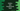# How to take Hexadecimal numbers as input in Python## How to take Hexadecimal numbers as input in Python:

This post will show you how to take hexadecimal values as user-inputs in Python. Hexadecimal is base 16 number system. So, in a hexadecimal input value, we can have any number from 1 to 9 or any of the letter A, B, C, D, E, F. That means, we will have to read the value as string for hexadecimal.

For example, hexadecimal representation of decimal 100 is 0X64.

We can also verify the data that is entered by the user, i.e. if it is a valid hexadecimal value or not.

In this post, we will learn how to take hexadecimal values as inputs from the user and how to verify if an input is hexadecimal or not.

## Take a hexadecimal number as input:

Since we can read a hexadecimal value as string, we can read it using input(). For example:

``````input_value = input("Enter a value: ")

print(input_value)``````

Here, we are reading the value as a string and storing it in the input_value variable. The last line is printing this value.

It will give output as like below:

``````Enter a value: 0x80
0x80``````

So, it is printing the same value that user has entered.

## Validate user input is hexadecimal or not:

We can also validate if the user input data is hexadecimal value or not. For that, we need to use int(). int() takes one second parameter. If we pass it as 16, it will try to parse the result as hexadecimal. If it is not hexadecimal, it will throw one ValueError. So, based on if it throws an error or not, we can say the input is a valid hexadecimal input or not.

``````input_value = input("Enter a value: ")

try:
int(input_value, 16)
except ValueError:
print("Please enter a valid number !")``````

As you can see here, we are using the try-except block to check if the input value is hexadecimal or not. If it throws an error, we are showing a message to enter a valid number to the user.

If you run this code, it will print one output as like below:

``````Enter a value: 9uul
Please enter a valid number !``````

As you can see here, we can take one hexadecimal value as an input in Python and we can keep that value in a string. But it is always a good idea to use a try-except block before storing the value in a variable.